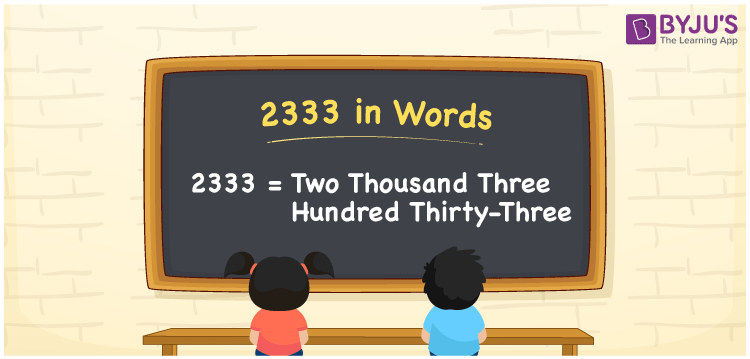# 2333 in Words

2333 in words is written as Two thousand three hundred thirty-three. In both the International System of Numerals and the Indian System of Numerals, 2333 is written as Two thousand three hundred thirty-three. The number 2333 is a Cardinal Number as it represents some quantity. For example, “the train tickets cost 2333 rupees”.

 2333 in Words Two thousand three hundred thirty-three Two thousand three hundred thirty-three in Number 2333

## 2333 in English Words

2333 in English words is read as “Two thousand three hundred thirty-three.”## How to Write 2333 in Words?

To write 2333 in words, we shall use the place value chart. In the place value chart, put 2 in the thousands, 3 in the hundreds, tens and ones, respectively. Let us make a place value chart to write the number 2333 in words.

 Thousands Hundreds Tens Ones 2 3 3 3

Thus, we can write the expanded form as

2 × Thousand + 3 × Hundred + 3 × Ten + 3 × One

= 2 × 1000 + 3 × 100 + 3 × 10 + 3 × 1

= 2000 + 300 + 30 + 3

= 2333

= Two thousand three hundred thirty-three.

2333 is the natural number that is succeeded by 2332 and preceded by 2334.

2333 in words – Two thousand three hundred thirty-three

• Is 2333 an odd number? – Yes
• Is 2333 an even number? – No
• Is 2333 a perfect square number? – No
• Is 2333 a perfect cube number? – No
• Is 2333 a prime number? – Yes
• Is 2333 a composite number? – No

## Frequently Asked Questions on 2333 in Words

Q1

### How to write 2333 in words?

2333 in words is written as Two thousand three hundred thirty-three.
Q2

### How to write 2333 in words in the International and Indian System of Numerals?

In both, the system of numerals, 2333 in words, is written as Two thousand three hundred thirty-three.
Q3

### How to write 2333 in a place value chart?

In the place value chart, write 2 in the thousands, 3 in the hundreds, tens and ones, respectively.